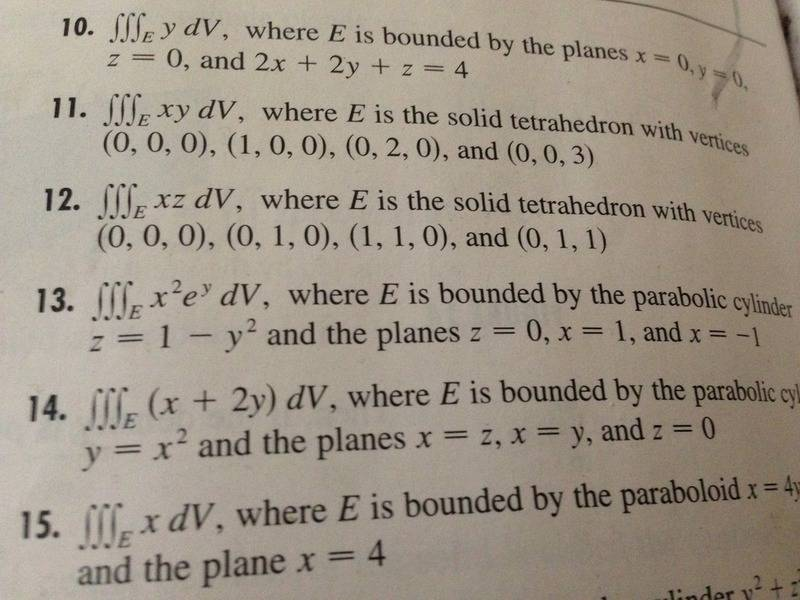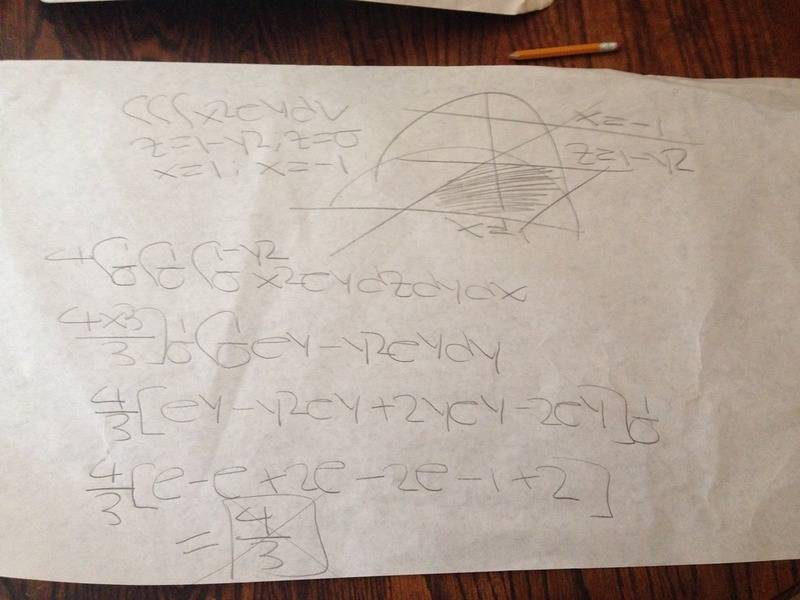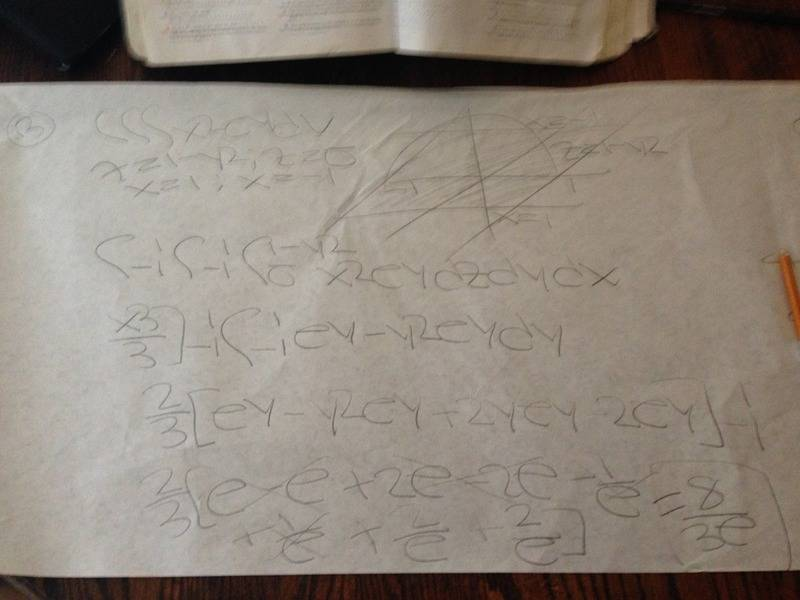# Triple Integral

Moved from a technical forum and thus no template
Here on problem #13 i am getting a false solution when only taking the volume of the region in quadrant 1 and multiplying it by 4. The later photo contains the correct solution which is obtained by taking the volume of the entire region. Can someone explain to me why the former is incorrect.LCKurtz
Homework Helper
Gold Member
Here on problem #13 i am getting a false solution when only taking the volume of the region in quadrant 1 and multiplying it by 4. The later photo contains the correct solution which is obtained by taking the volume of the entire region. Can someone explain to me why the former is incorrect.
Just because you are calculating a triple integral, it doesn't mean you are calculating a volume. If the integrand was ##1##, you would be, and your method would be correct. But your integrand is ##x^2e^y##, and ##e^y## is not symmetric about ##y=0##. So you don't get the same answer for each quadrant.

Ray Vickson
Homework Helper
Dearly Missed
Here on problem #13 i am getting a false solution when only taking the volume of the region in quadrant 1 and multiplying it by 4. The later photo contains the correct solution which is obtained by taking the volume of the entire region. Can someone explain to me why the former is incorrect.
View attachment 204190
View attachment 204191
View attachment 204192

I tried the problem myself. Is anyone else getting ##\frac{8}{3}e^{-1}##?

LCKurtz